### Nanopore data

Here at the Simpsonlab we work with signal-level data from nanopore sequencers, particularly those from ONT like the MinION. Signal-level data looks something like this: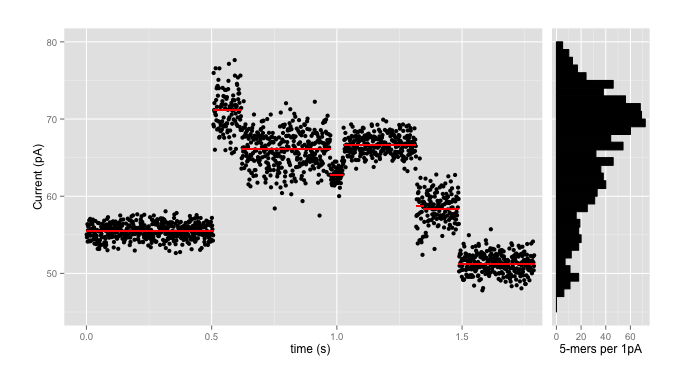where the data comes in as a stream of events (the red lines) with means, standard deviations, and durations, with each black point being an individual signal reading. We use the signal-level data partly for accuracy - because converting each red line and dozens of black points into one of four letters invariably loses lots of information - and partly because those basecalls might not be available in, say, the field, where the portability of the nanopore sequencers really shines.

This data has to be interpreted in terms of a particular model of the interaction of the pore and the strand of DNA; such a pore model gives, amongst other things, an expected signal mean and standard deviation for currents through the pore when a given $$k$$mer is in the pore (for the MinION devices, pore models typically use $$k$$=5 or 6). So a pore model might be, for $$k$$=5, a table with 1024 entries that looks something like this:

kmer mean std dev
AAAAA 70.24 ± 0.95 pA
AAAAC 66.13 ± 0.75 pA
AAAAG 70.23 ± 0.76 pA
AAAAT 69.25 ± 0.68 pA

and using such a pore model it’s fairly straightforward to go from a sequence to an expected set of signal levels from the sequencer: so, say, for the 10-base sequence AAAAACGTCC and the above 5-mer pore model we’d expect 6 events (10-5+1) that look like:

event kmer mean range (1$$\sigma$$)
e1 AAAAA 70.2 pA 69.3 - 71.2 pA
e2 AAAAC 66.1 pA 65.4 - 66.9 pA
e3 AAACG 62.8 pA 62.0 - 63.6 pA
e4 AACGT 67.6 pA 66.3 - 68.9 pA
e5 ACGTC 56.6 pA 54.5 - 58.7 pA
e6 CGTCC 49.9 pA 49.1 - 50.7 pA

Using those models, plus HMMs and a tonne of math, Jared’s nanopolish tool has had enormous success improving the quality of nanopore reads and assemblies of nanopore data. As one can see from the ‘range’ column above, and from the histogram on the side of the first plot, which shows the numbers of kmers which fall into 1pA bins in the pore model, this isn’t necessarily particularly easy. There are over 70 kmers which are expected to have means in the range 69.5pA - 70.5pA; that plus the wide standard deviations means that there is enormous ambiguity going back from signal levels to sequence.

### Mapping

This ambiguity introduces complication into another part of the bioinformatics pipeline - mapping. While several mappers (BWA MEM, LAST) work quite well with basecalled ONT data, and one mapper1 has been written specifically for basecalled ONT data, mapping signal-level data directly is a little tougher. It is one thing for a seed sequence in the read to occur in multiple locations in the reference index; it is another thing for a sequence of floating-point currents to plausibly represent one of hundreds of seed sequences.

On the other hand, a flurry of recent important papers and software2 3 4 5, the first of which are discussed in some informative blog posts, have demonstrated the usefulness of approximate read mapping. In our case, mapping to an approximate region of a reference would allow exact but computationally expensive methods like nanopolish eventalign to produce precise alignments, or simple assignment may be all that is necessary for other applications.

So an output approximate mapping would be valuable, but the issues remains of how to disambiguate the signal level values.

### Spatial Indexing

Importantly, while any individual event is very difficult to assign with precision, sequences of events are less ambiguous, as any given event is followed, with high probability, by one of only four other events. Thus, by examining tuples of events, one can greatly increase specificity.

And there are well-developed sets of techniques for looking up lists of $$d$$ floating point values to look for candidate matches: spatial indexes, where each query or match is considered as a point in $$d$$-dimensional space, and the goal is to return either some number of nearest points (k-Nearest-Neighbours, or kNN queries), or all matches within some, typically euclidean, distance.

To see how this would work, consider indexing the sequence above, AAAAACGTCC, in a spatial index with $$d=2$$. There are a total of 6 events, so we’d have 5 points (6-2+1), each points in 2-dimensional space; 4 are plotted below (the other falls out of the range of the plot)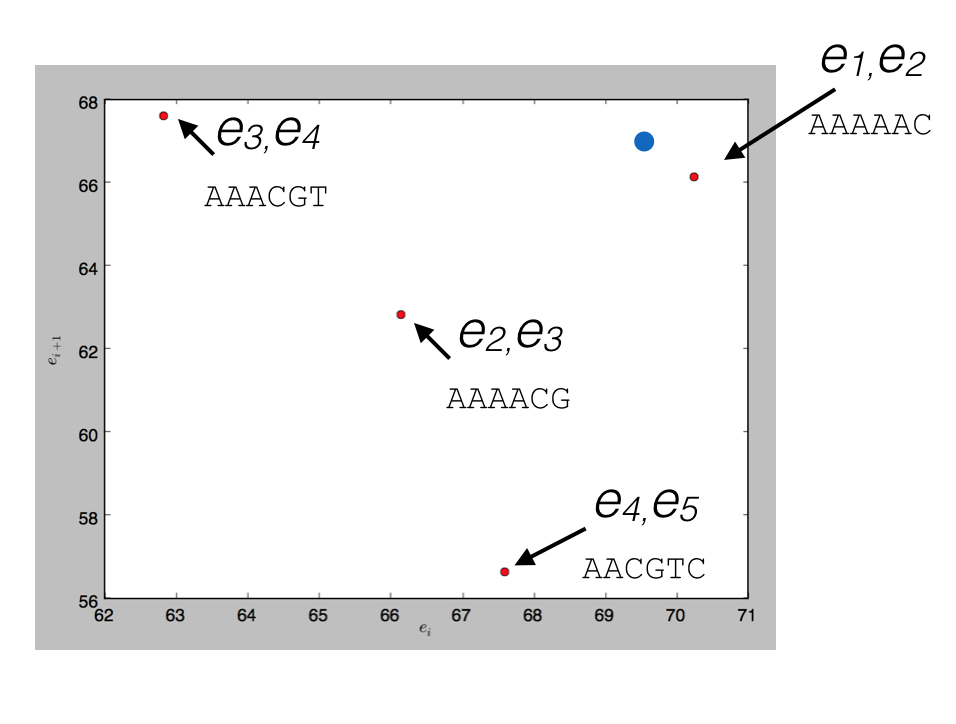and a query for $$d$$ read events that could be matched by (69.5, 67), the blue point, would return the nearest match (70.2, 66.1) corresponding to the $$k+d-1$$-mer AAAAAC.

So to map event data, one can:

• Generate an index:
• Generate all overlapping tuples of $$d$$ kmers from a reference
• Using a pore model, convert those into points in $$d$$-space
• Create a spatial index of those points,
• Take every overlapping set of $$d$$ events
• Look it up in a spatial index
• Find a most likely starting point for the read in the reference, with a quality score.

Note that one has to explicitly index both the forward and reverse strands of the reference, since you don’t a priori know what the “reverse complement” of 65.5 pA is. One also has to generate multiple indices; for 2D ONT reads, there is a pore model for the template strand of a read, and typically two possible models to choose from for the complement strand of a read, so one needs three indexes in total to query.

### What dimension to choose?

To test this approach, you have to choose a $$d$$ to use. On the one hand, you would like to choose as large a dimension as you can; the larger $$k+d-1$$ is, the more unique each seed is and the fewer places it will occur in any reference sequence.

On the other hand, two quite different constraints put a strong upper limit on the dimensions that will be useful:

• The curse of dimensionality - in high spatial dimensions, nearest-neighbour searching is extremely inefficient, because distance loses its discriminative power. (Most things are roughly equally far from each other in 100-dimensional space; there are a lot of routes you can take!) As a practical matter, for most spatial index implementations, for $$d > 15-20$$ or so you might as well just do a linear search over all possible items;
• Current approaches to segmentation mean that ONT data has very frequent ‘stops’ and ‘skips’ - that is, events are spuriously either inserted or deleted from the data. Exactly as with noisy basecalled sequences, this strongly limits the length of sequence that can be usefully used as seeds. As we see below for one set of E. coli data, there is probably not much point in using $$d \ge 10$$ even for template-strand data, as the median “dmer” will have an insertion/deletion in it.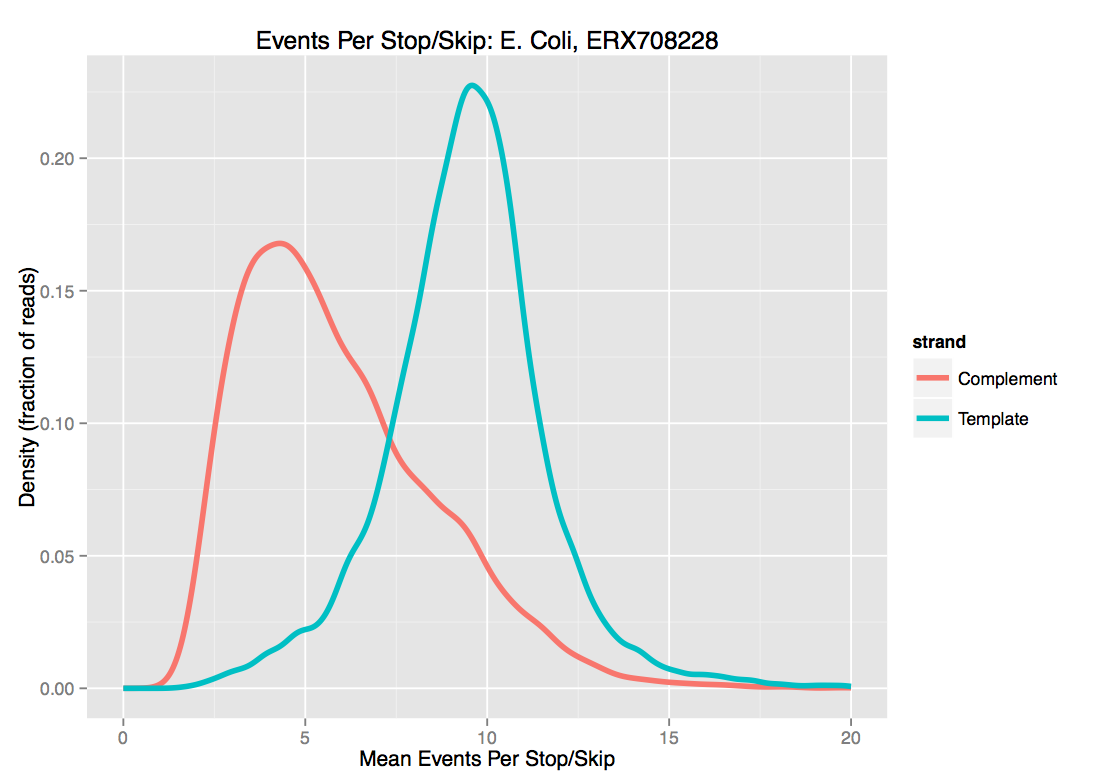For these two reasons, we’ve been playing with $$d \approx 8$$. I’ll note that while increasing $$d$$ is the most obvious knob to turn to increase specificity of the match, higher $$k$$ helps as well.

### Normalizing the signal levels

One issue we haven’t mentioned is that the raw nanopore data needs calibration to be compared to the model; there is an additive shift and drift over time, and a multiplicative scale that has to be taken into account.

A very simple “methods of moments” calculation works surprisingly well for long-enough reads, certainly well enough to start an iterative process; for any given model one is trying to fit a read to, rescaling the mean and standard deviation of read events to model events gives a good starting point for calibration, and drift is initially ignored.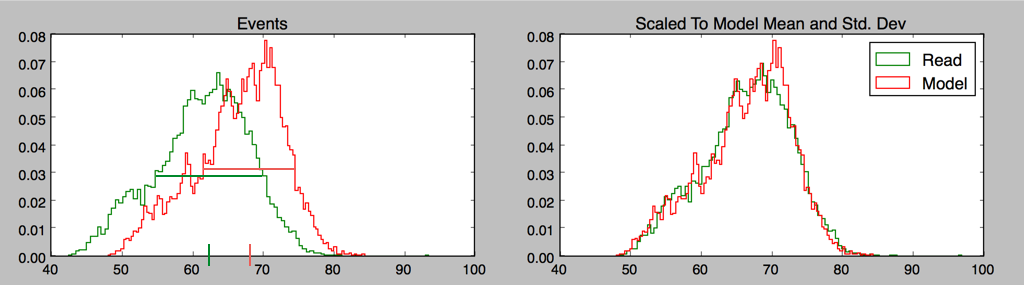### Proof of concept

A simple proof of concept of using spatial indexing to approximately map squiggle data can be found on github. It’s written in python, and has scipy, h5py, and matplotlib as dependencies. Note that as implemented, it is absurdly memory-hungry, and definitely requires a numpy and scipy built against a good BLAS implementation.

As a spatial index, it uses a version of a k-d tree (scipy.spatial.cKDTree), which is a very versatile and widely used (and so well-optimized) spatial index widely used in machine learning methods amongst others; different structures may have advantages for this application.

Running the index-and-map.sh script generates an index for the provided ecoli.fa reference - about 1 minute per pore model - and then maps the 10 reads provided of both older 5mer and newer 6mer MAP data. Mapping each read takes about 6 seconds per read per pore model; this involves lots of python list manipulations so could fairly straightforwardly be made much faster.
Doing the simplest thing possible for mapping works surprisingly well. Using the same sort of approach as the first steps of the Sovic et al. method1, we just:

• Use the default k-d tree parameters (which almost certainly isn’t right, particularly the distance measure)
• Consider overlapping bins of starting positions on the reference, of size ~10,000 events, a typical read size
• For each $$d$$-point in the read,
• Take the closest match to each $$d$$-point (or all within some distance)
• For each match, add a score to the bin corresponding to the implied starting position of the read on the reference; a higher score for a closer match
• Report the best match starting point

Let’s take a look at the initial output for the older SQK005 ecoli data and newer SQK006 data:

We see a couple of things here:

• Adding the complement strand does almost nothing for the accuracy, but requires substantially more memory and compute time, as multiple indices must be loaded and used up, and all candidate complement indices must be compared against each other. Because of this and the typically higher skip/stay rates for complement strands, we will use the template strand only for the rest of this post;
• Since we are simply assigning starting bins at this point, any assignments within the bin size are equally accurate; here, all of the reads were correctly assigned to the starting bin or the neighbouring one.
• The newer 6mer data gives slightly better results; part of this is likely because we are using the same $$d$$, so a dmer for the k=6 data corresponds to seed longer by one
• The zscore here is a very crude measure of how much the assignment stands out over the background (but not necessarily how it compares to other candidate mappings); some of these very simple pseudo-mappings are relatively securely identified, and others less so. The sum-of-scores for the reads with the best and worst zscore results are plotted below; no prize for guessing which is which:
 5mer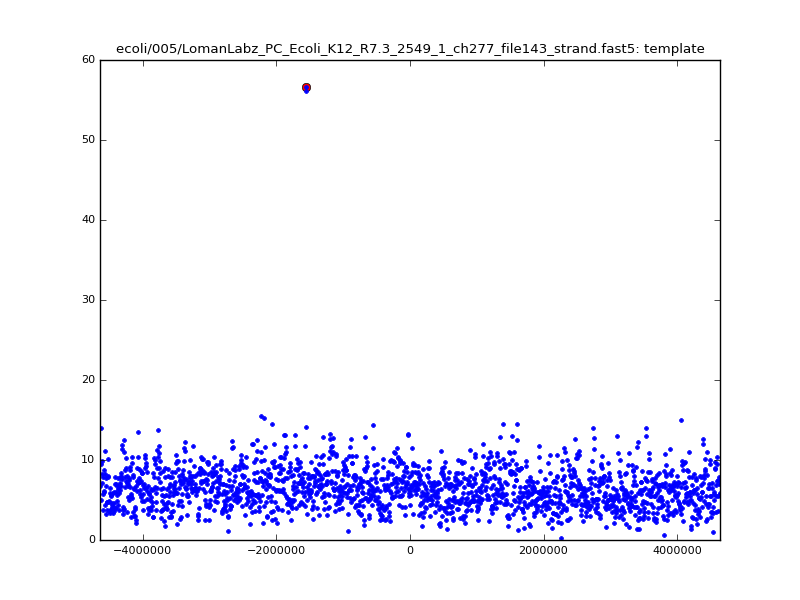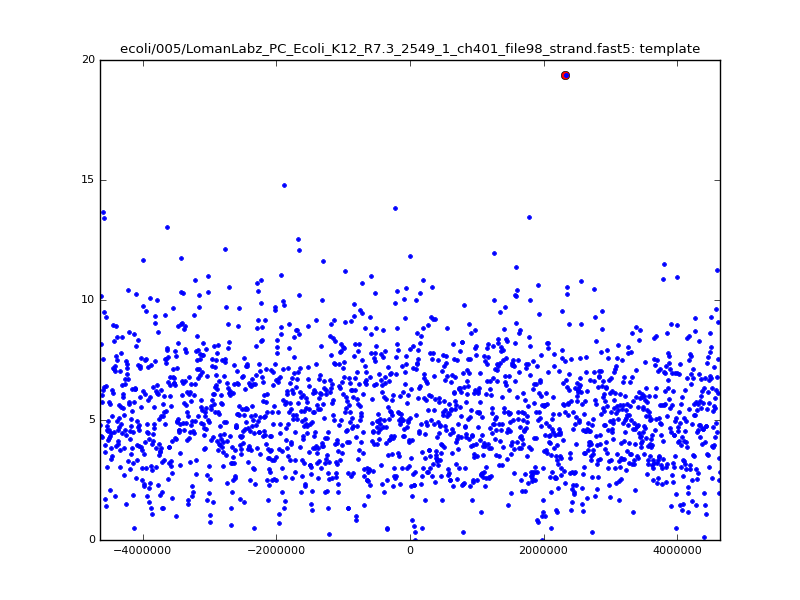6mer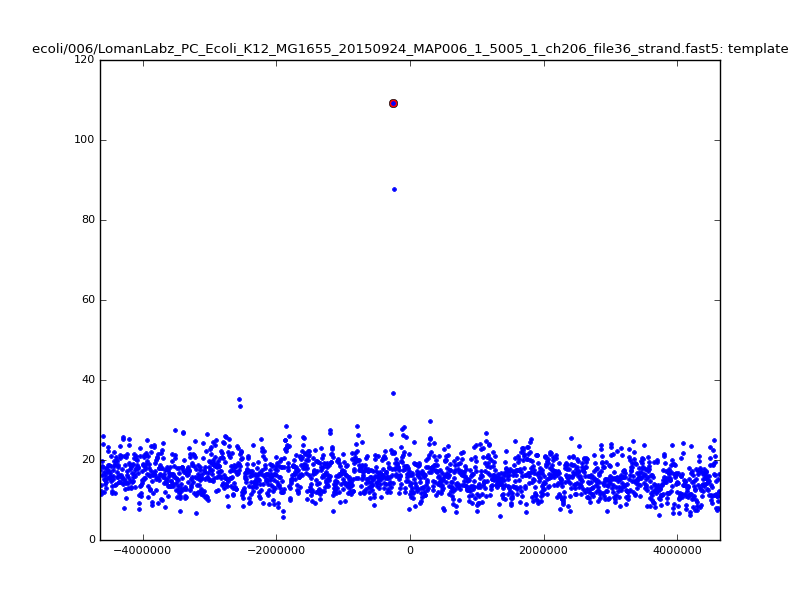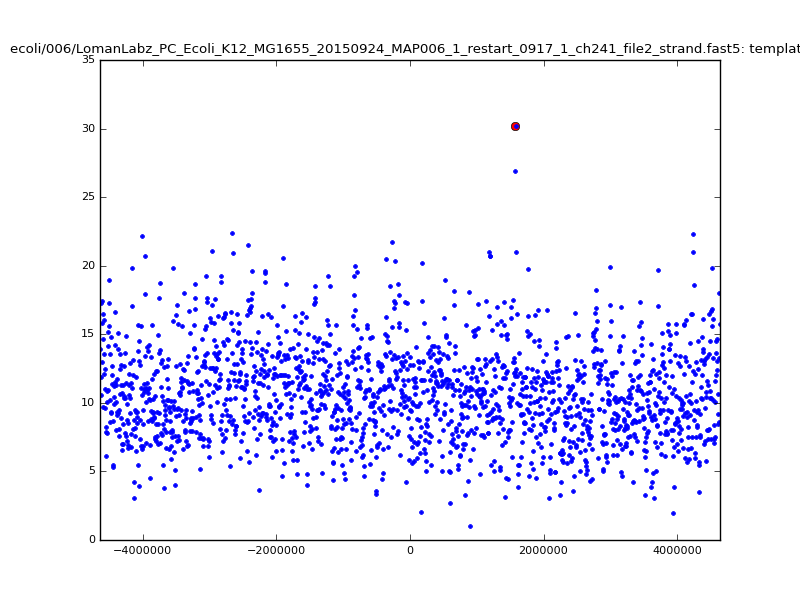Because many levels are clustered near ~60-70pA, many dmers are quite close to each other, and choosing simply the closest $$d$$-point is unlikely to give a robust result. Examining all possible matches in the spatial index within some given radius reduces the noise somewhat, at a modest increase in compute time:

Note the increase in zscores; the same two reads are plotted:

 5mer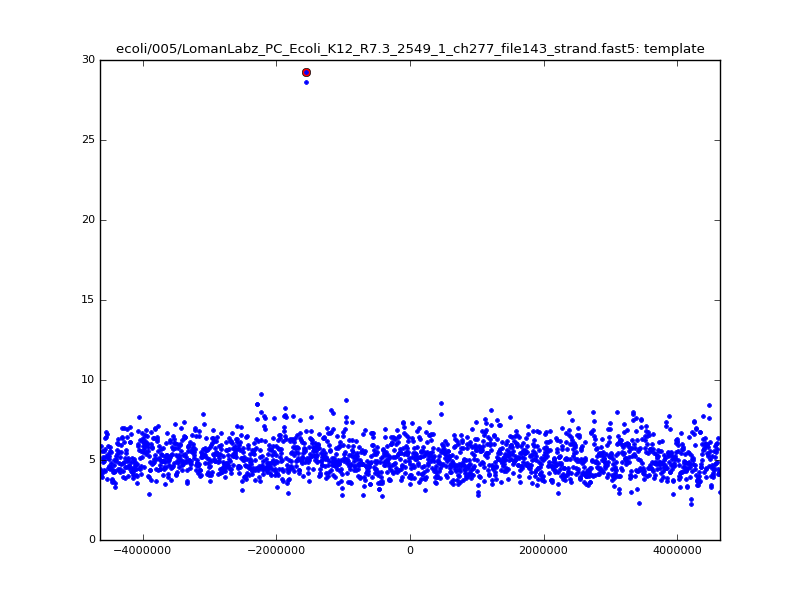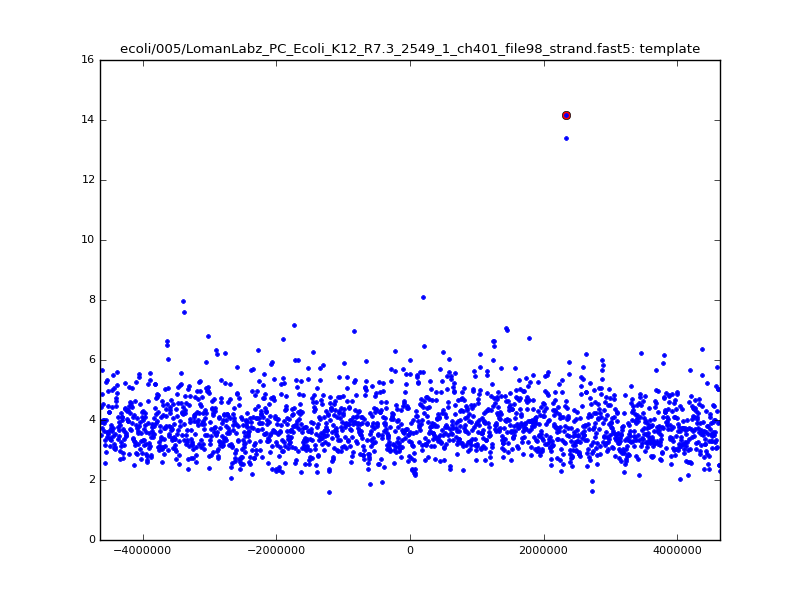6mer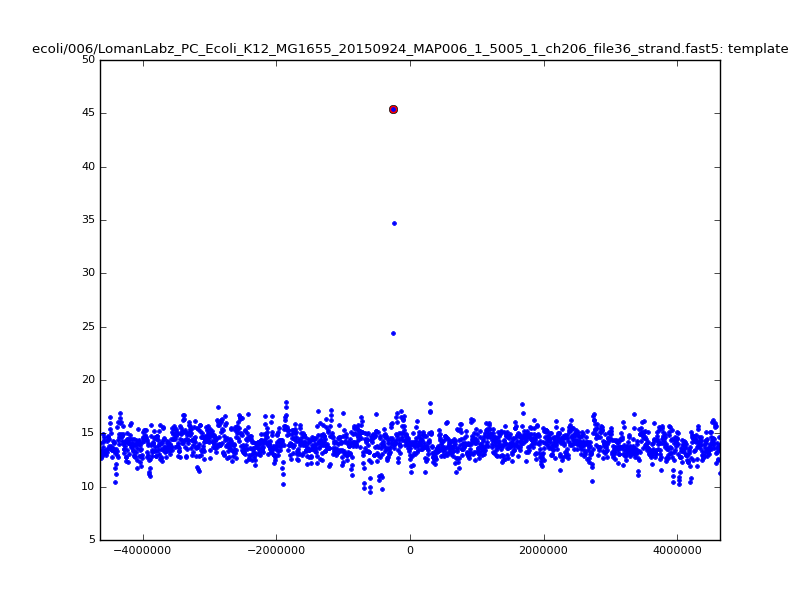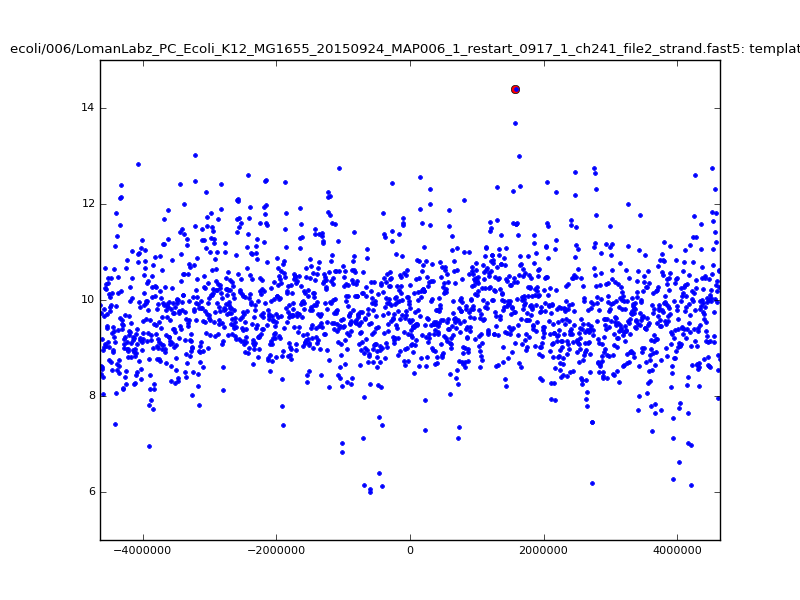With a few other tweaks - keeping track of the top 10 candidates, and for each re-testing by recalibrating given the inferred mapping and rescoring - we can get about 95%-99% accuracy in mapping.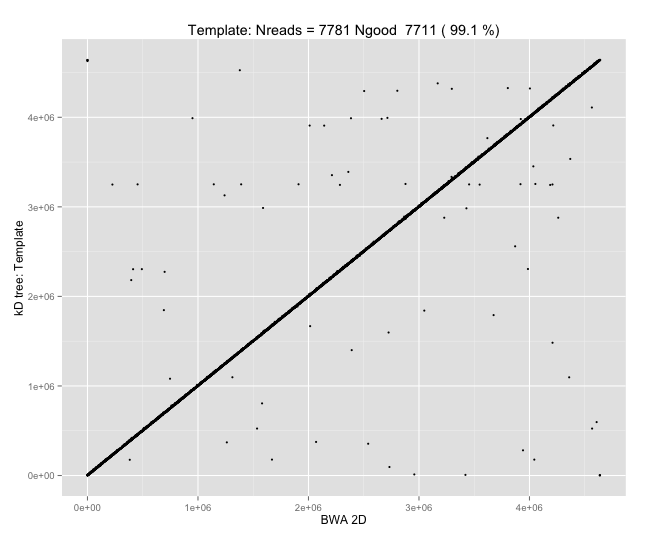Of course, while 95-99% (Q13-Q20) mapping accuracy on E. coli is a cute outcome from such a simple approach, it isn’t nearly enough; with $$d=8$$ and $$k=5$$, wer’e working with seeds of size 12, which would typically be unique in the E. coli reference, but certainly wouldn’t be in the human genome, or for metagenomic applications.

### EM Rescaling

One limiting factor is the approximate nature of the rescaling so far that is being performed; for reads that have been basecalled, the inferred shift values above can be off by several picoamps from the Metrichor values, which clearly causes both false negatives and false positives in the index lookup. This can be addressed by doing a more careful rescaling step, using EM iterations:

• For the E-step, provisionally assign probabilities of read events corresponding to model levels, based on the Gaussian distributions described in the model and a simple transition matrix between events;
• For the M-step, perform a weighted least squares regression to re-scale the read levels.

This gives answers that are quite good when compared with Metrichor, at the cost of substantially more computational effort (much greater than the spatial index lookup!), particularly for long reads and larger (k) where the number of model levels is larger:

Note again the increase in zscores; replotting gives:

 5mer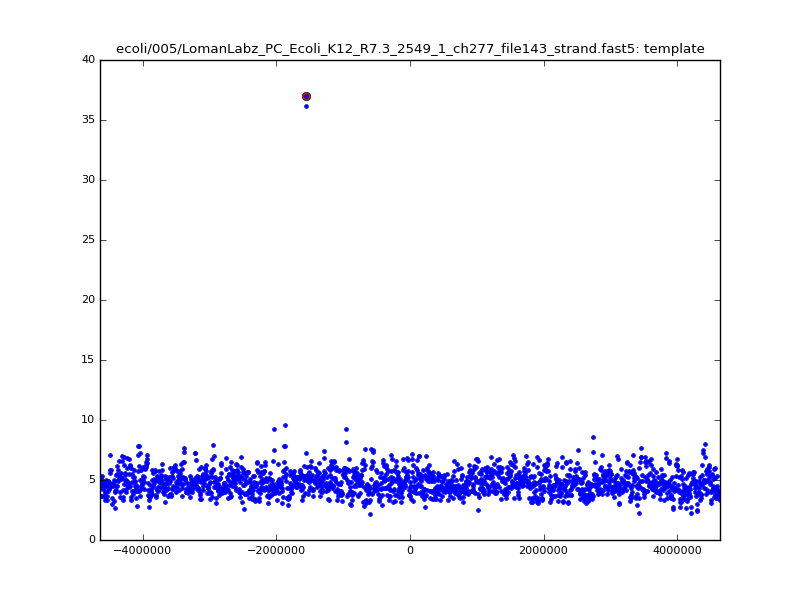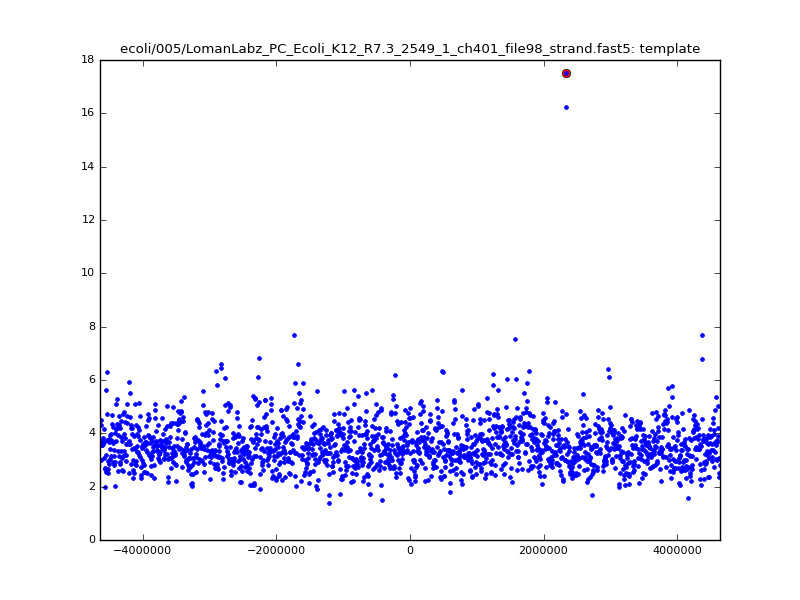6mer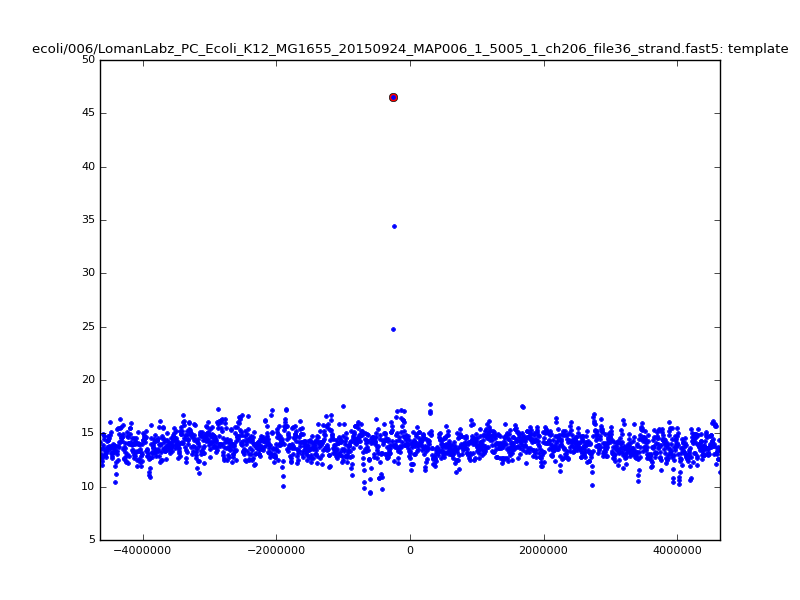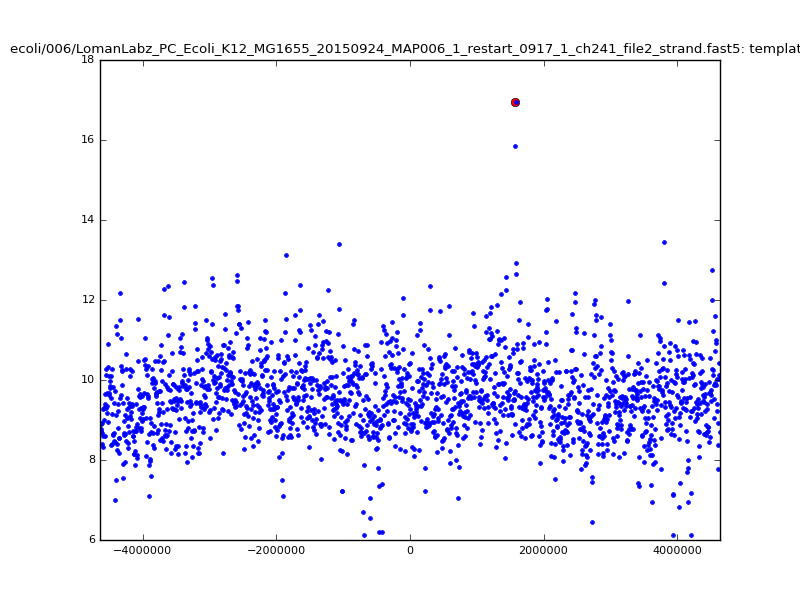### Extending the seeds

So far we have in no way taken into account any of the locality information in the spatial index lookup results; that is, that a long series of hits close together, in the same order on the read and on the reference, is much stronger evidence for a good mapping than a haphazard series of hits in random order that happen to fall within the same bin of starting points.

Keeping with the do-the-simplest-thing approach that has worked so far, we can try to extend these “seed” matches by stitching them together into longer seeds; here we build 15-mers out of sets of 4 neighbouring 12-mers, allowing one skip or stay somewhere within them, using as a score for the result the minimum of the constituent scores, and dropping all hits that cannot be so extended. This has quite modest additional cost, and works quite well:

 5mer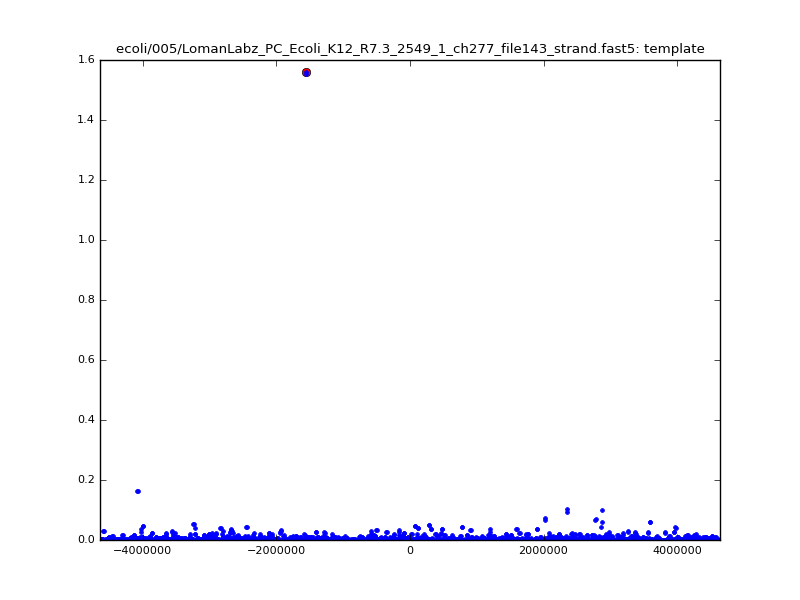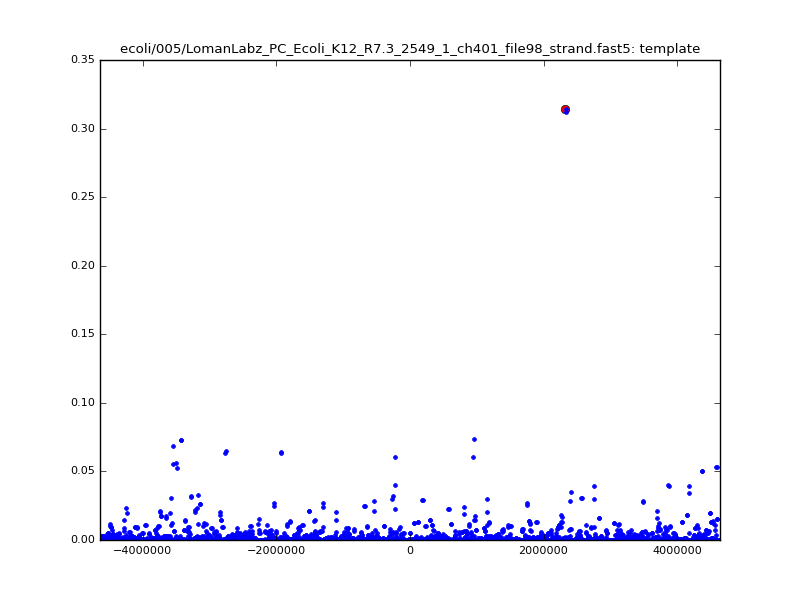6mer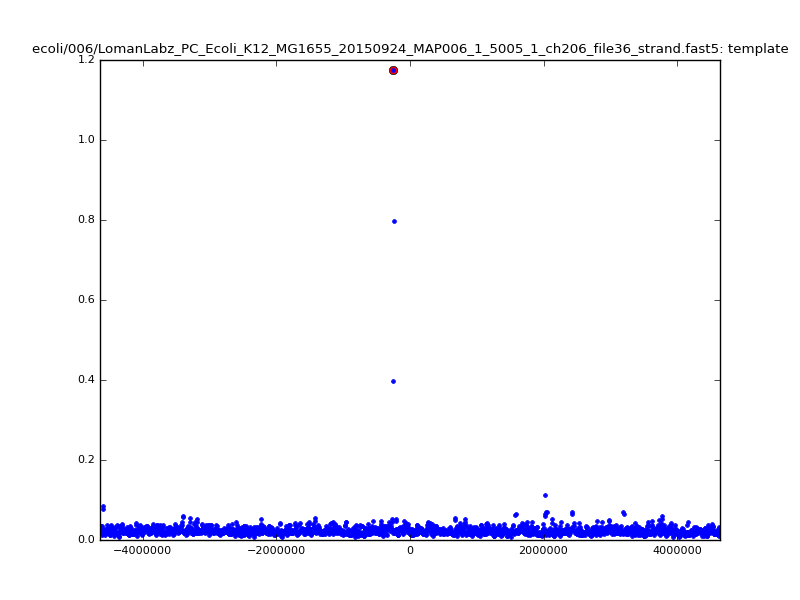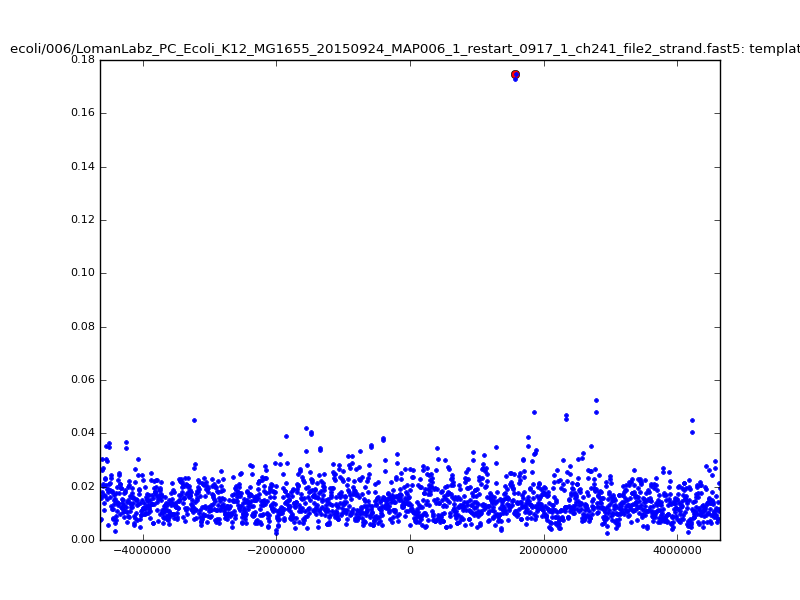Now that we seem to have much stronger and more specific results, we can investigate letting go of binning, and instead string these extended seeds into longest possible matches. Continuing to extend dmer-by-dmer is too challenging due to missing points (due to mis-calibration, too large noise in the dmer falling out of the maxdist window in the kdtree, or several skip/stays in a row), so following the ideas of graphmap1 and minimap5, we trace collinear seeds through the set of available seeds; rather than just taking longest increasing sequences in inferred start positions, however, we make a graph of seeds with edges connecting seeds that are monotonically increasing both in read location and reference location with ‘small enough’ jumps, and extract longest paths through this graph. The cost of this is smaller than the rescaling of the read.

Running this, the zscores now somewhat change meaning; only the extracted paths are scored, meaning that the scores are only amongst plausible mappings, not over all bins across the reference. Similarly, the differences in mapping locations are somewhat more meaningful - rather than being compared to bin centres, they are actually the differences between mapping locations.

We get:

 5mer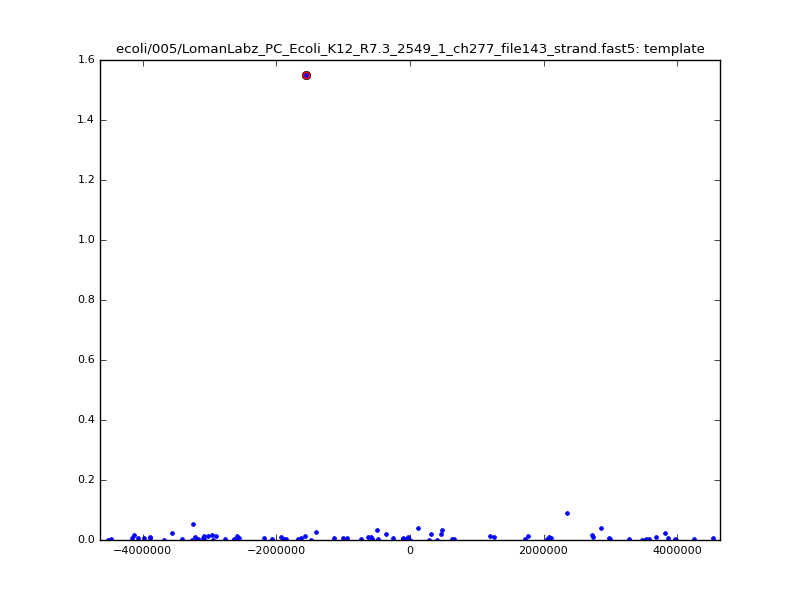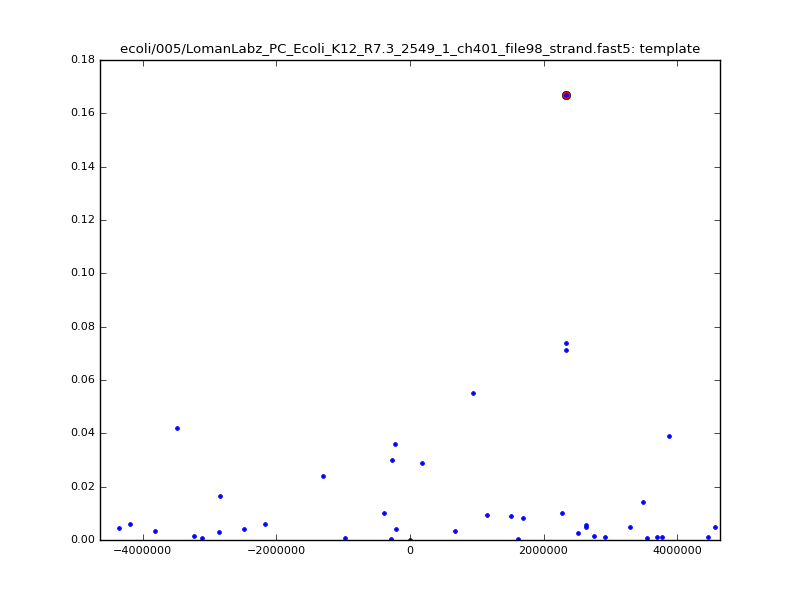6mer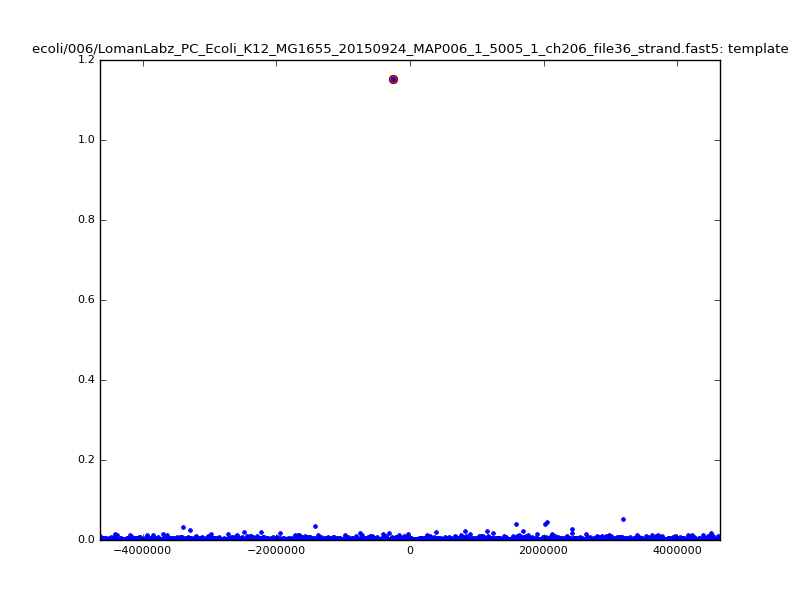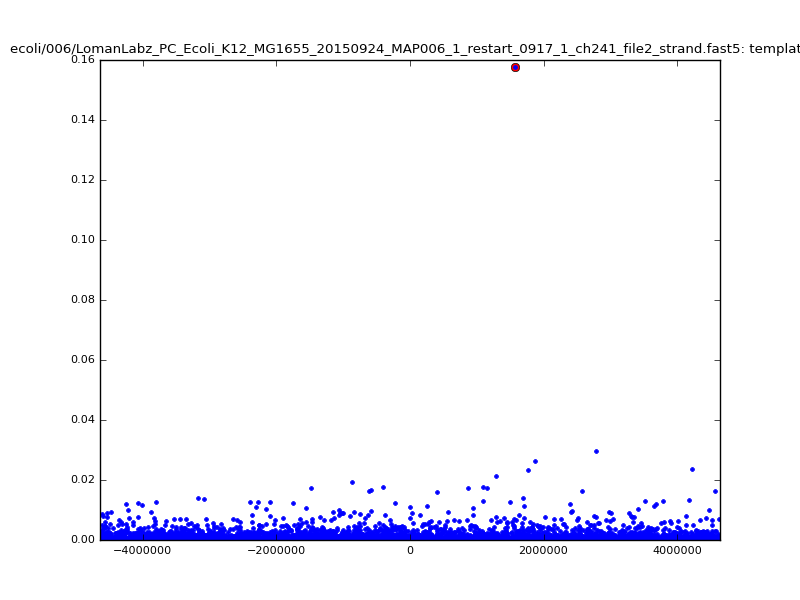The simple python testbed implementation of these ideas linked to above is very slow, single-threaded, absurdly memory hungry, and its treatment of scores for the mappings does not currently make much sense. In the new year we will address these issues in a proper C++ implementation, where (for instance) we will not have multiple copies of large, reference-sized data structures.